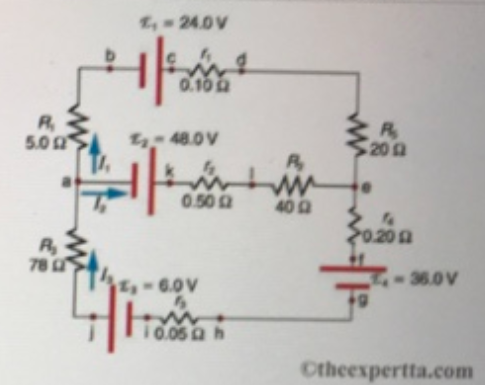# Problem: Consider the currents in the figureWhat equation do you get when you apply Kirchhoff’s junction rule at point a?

###### FREE Expert Solution

According to Kirchhoff's junction rule, the currents entering a junction is equal to the currents leaving the junction.

92% (181 ratings)###### Problem Details

Consider the currents in the figureWhat equation do you get when you apply Kirchhoff’s junction rule at point a?